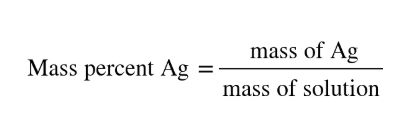# Problem: Silver nitrate solutions are often used to plate silver onto other metals.What is the maximum amount of silver (in grams) that can be plated out of 4.8 L of an AgNO3 solution containing 3.4 % Ag by mass? Assume that the density of the solution is 1.01 g/mL.

###### FREE Expert Solution
• Mass percent Ag can be written as:• This means that if we have 3.4% Ag, we can assume that there are 3.4 g Ag in every 100 g of AgNO3

99% (439 ratings)###### Problem Details

Silver nitrate solutions are often used to plate silver onto other metals.

What is the maximum amount of silver (in grams) that can be plated out of 4.8 L of an AgNO3 solution containing 3.4 % Ag by mass? Assume that the density of the solution is 1.01 g/mL.